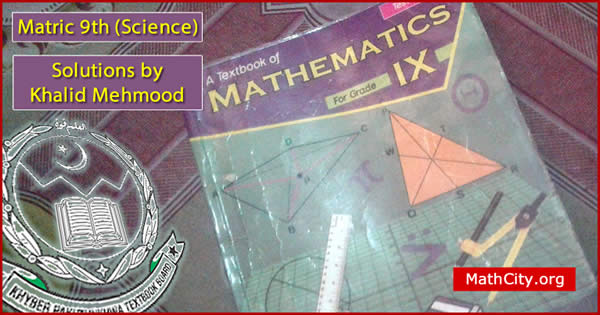# Mathematics 9 (Science) - KPKSolutions of the Textbook of Mathematics for Grade IX, published by The Khyber Pakhtunkhwa Textbook Board, Peshawar, Pakistan are given here. These notes are send by Khalid Mehmood. We are very thankful to him for sending theses notes. There are seventeen (17) chapters in this book.

• Chapter 1: Matrices and Determinants
• Chapter 2: Real and Complex Numbers
• Chapter 3: Logarithms
• Chapter 4: Algebraic Expressions and Algebraic
• Chapter 5: Factorization
• Chapter 6: Algebraic Manipulation
• Chapter 7: Linear Equations and Inequalities
• Chapter 8: Linear Graphs & Their Applications
• Chapter 9: Introduction to Coordinate Geometry
• Chapter 10: Descriptive Geometry Congruent Triagles
• Chapter 10-16: Theorems
• Chapter 17: Practical Geometry
• matric/kpk-9th-science